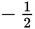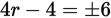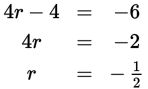# SAT Math Multiple Choice Question 932: Answer and Explanation

### Test Information

Question: 932

16. If r < 0 and (4r – 4)2 = 36, what is the value of r?

• A. –6
• B. –2
• C. –2
• D.Explanation:

D

Difficulty: Medium

Getting to the Answer: Take the square root of both sides of the equation to remove the exponent and get. You're looking for a value of r that is less than 0, so start by solving the equation with -6: